# Simulating a single compartment Hodgkin-Huxley neuron#

In this section we will model and simulate a Hodgkin-Huxley (HH) neuron ([HH52]). A Hodgkin-Huxley neuron includes Sodium (Na), Potassium (K), and leak ion channels. For further information on this neuron model, please see here.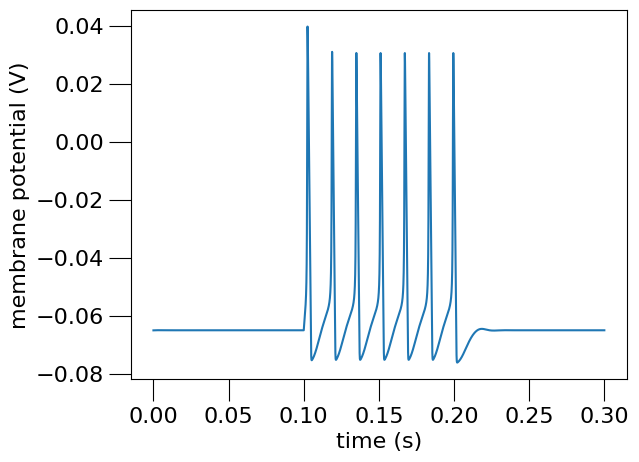Fig. 13 Membrane potential of the simulated Hodgkin-Huxley neuron.#

This plot, saved as HH_single_compartment_example_sim-v.png is generated using the following Python NeuroML script:

#!/usr/bin/env python3
"""
Create a network with a single HH cell, and simulate it.

File: hh-single-compartment.py

Copyright 2021 NeuroML contributors
Author: Ankur Sinha <sanjay DOT ankur AT gmail DOT com>
"""

import math
from neuroml import NeuroMLDocument
from neuroml import Cell
from neuroml import IonChannelHH
from neuroml import GateHHRates
from neuroml import BiophysicalProperties
from neuroml import MembraneProperties
from neuroml import ChannelDensity
from neuroml import HHRate
from neuroml import SpikeThresh
from neuroml import SpecificCapacitance
from neuroml import InitMembPotential
from neuroml import IntracellularProperties
from neuroml import IncludeType
from neuroml import Resistivity
from neuroml import Morphology, Segment, Point3DWithDiam
from neuroml import Network, Population
from neuroml import PulseGenerator, ExplicitInput
import numpy as np
from pyneuroml import pynml
from pyneuroml.lems import LEMSSimulation

def main():
"""Main function

Include the NeuroML model into a LEMS simulation file, run it, plot some
data.
"""
# Simulation bits
sim_id = "HH_single_compartment_example_sim"
simulation = LEMSSimulation(sim_id=sim_id, duration=300, dt=0.01, simulation_seed=123)
# Include the NeuroML model file
simulation.include_neuroml2_file(create_network())
# Assign target for the simulation
simulation.assign_simulation_target("single_hh_cell_network")

# Recording information from the simulation
simulation.create_output_file(id="output0", file_name=sim_id + ".dat")

# Save LEMS simulation to file
sim_file = simulation.save_to_file()

# Run the simulation using the default jNeuroML simulator
pynml.run_lems_with_jneuroml(sim_file, max_memory="2G", nogui=True, plot=False)
# Plot the data
plot_data(sim_id)

def plot_data(sim_id):
"""Plot the sim data.

Load the data from the file and plot the graph for the membrane potential
using the pynml generate_plot utility function.

:sim_id: ID of simulaton

"""
data_array = np.loadtxt(sim_id + ".dat")
pynml.generate_plot([data_array[:, 0]], [data_array[:, 1]], "Membrane potential", show_plot_already=False, save_figure_to=sim_id + "-v.png", xaxis="time (s)", yaxis="membrane potential (V)")
pynml.generate_plot([data_array[:, 0]], [data_array[:, 2]], "channel current", show_plot_already=False, save_figure_to=sim_id + "-i.png", xaxis="time (s)", yaxis="channel current (A)")
pynml.generate_plot([data_array[:, 0], data_array[:, 0]], [data_array[:, 3], data_array[:, 4]], "current density", labels=["Na", "K"], show_plot_already=False, save_figure_to=sim_id + "-iden.png", xaxis="time (s)", yaxis="current density (A_per_m2)")

def create_na_channel():
"""Create the Na channel.

This will create the Na channel and save it to a file.
It will also validate this file.

returns: name of the created file
"""
na_channel = IonChannelHH(id="na_channel", notes="Sodium channel for HH cell", conductance="10pS", species="na")
gate_m = GateHHRates(id="na_m", instances="3", notes="m gate for na channel")

m_forward_rate = HHRate(type="HHExpLinearRate", rate="1per_ms", midpoint="-40mV", scale="10mV")
m_reverse_rate = HHRate(type="HHExpRate", rate="4per_ms", midpoint="-65mV", scale="-18mV")
gate_m.forward_rate = m_forward_rate
gate_m.reverse_rate = m_reverse_rate
na_channel.gate_hh_rates.append(gate_m)

gate_h = GateHHRates(id="na_h", instances="1", notes="h gate for na channel")
h_forward_rate = HHRate(type="HHExpRate", rate="0.07per_ms", midpoint="-65mV", scale="-20mV")
h_reverse_rate = HHRate(type="HHSigmoidRate", rate="1per_ms", midpoint="-35mV", scale="10mV")
gate_h.forward_rate = h_forward_rate
gate_h.reverse_rate = h_reverse_rate
na_channel.gate_hh_rates.append(gate_h)

na_channel_doc = NeuroMLDocument(id="na_channel", notes="Na channel for HH neuron")
na_channel_fn = "HH_example_na_channel.nml"
na_channel_doc.ion_channel_hhs.append(na_channel)

pynml.write_neuroml2_file(nml2_doc=na_channel_doc, nml2_file_name=na_channel_fn, validate=True)

return na_channel_fn

def create_k_channel():
"""Create the K channel

This will create the K channel and save it to a file.
It will also validate this file.

:returns: name of the K channel file
"""
k_channel = IonChannelHH(id="k_channel", notes="Potassium channel for HH cell", conductance="10pS", species="k")
gate_n = GateHHRates(id="k_n", instances="4", notes="n gate for k channel")
n_forward_rate = HHRate(type="HHExpLinearRate", rate="0.1per_ms", midpoint="-55mV", scale="10mV")
n_reverse_rate = HHRate(type="HHExpRate", rate="0.125per_ms", midpoint="-65mV", scale="-80mV")
gate_n.forward_rate = n_forward_rate
gate_n.reverse_rate = n_reverse_rate
k_channel.gate_hh_rates.append(gate_n)

k_channel_doc = NeuroMLDocument(id="k_channel", notes="k channel for HH neuron")
k_channel_fn = "HH_example_k_channel.nml"
k_channel_doc.ion_channel_hhs.append(k_channel)

pynml.write_neuroml2_file(nml2_doc=k_channel_doc, nml2_file_name=k_channel_fn, validate=True)

return k_channel_fn

def create_leak_channel():
"""Create a leak channel

This will create the leak channel and save it to a file.
It will also validate this file.

:returns: name of leak channel nml file
"""
leak_channel = IonChannelHH(id="leak_channel", conductance="10pS", notes="Leak conductance")
leak_channel_doc = NeuroMLDocument(id="leak_channel", notes="leak channel for HH neuron")
leak_channel_fn = "HH_example_leak_channel.nml"
leak_channel_doc.ion_channel_hhs.append(leak_channel)

pynml.write_neuroml2_file(nml2_doc=leak_channel_doc, nml2_file_name=leak_channel_fn, validate=True)

return leak_channel_fn

def create_cell():
"""Create the cell.

:returns: name of the cell nml file
"""
# Create the nml file and add the ion channels
hh_cell_doc = NeuroMLDocument(id="cell", notes="HH cell")
hh_cell_fn = "HH_example_cell.nml"
hh_cell_doc.includes.append(IncludeType(href=create_na_channel()))
hh_cell_doc.includes.append(IncludeType(href=create_k_channel()))
hh_cell_doc.includes.append(IncludeType(href=create_leak_channel()))

# Define a cell
hh_cell = Cell(id="hh_cell", notes="A single compartment HH cell")

# Define its biophysical properties
bio_prop = BiophysicalProperties(id="hh_b_prop")
#  notes="Biophysical properties for HH cell")

# Membrane properties are a type of biophysical properties
mem_prop = MembraneProperties()
# Add membrane properties to the biophysical properties
bio_prop.membrane_properties = mem_prop

# Append to cell
hh_cell.biophysical_properties = bio_prop

# Channel density for Na channel
na_channel_density = ChannelDensity(id="na_channels", cond_density="120.0 mS_per_cm2", erev="50.0 mV", ion="na", ion_channel="na_channel")
mem_prop.channel_densities.append(na_channel_density)

# Channel density for k channel
k_channel_density = ChannelDensity(id="k_channels", cond_density="360 S_per_m2", erev="-77mV", ion="k", ion_channel="k_channel")
mem_prop.channel_densities.append(k_channel_density)

# Leak channel
leak_channel_density = ChannelDensity(id="leak_channels", cond_density="3.0 S_per_m2", erev="-54.3mV", ion="non_specific", ion_channel="leak_channel")
mem_prop.channel_densities.append(leak_channel_density)

# Other membrane properties
mem_prop.spike_threshes.append(SpikeThresh(value="-20mV"))
mem_prop.specific_capacitances.append(SpecificCapacitance(value="1.0 uF_per_cm2"))
mem_prop.init_memb_potentials.append(InitMembPotential(value="-65mV"))

intra_prop = IntracellularProperties()
intra_prop.resistivities.append(Resistivity(value="0.03 kohm_cm"))

# Add to biological properties
bio_prop.intracellular_properties = intra_prop

# Morphology
morph = Morphology(id="hh_cell_morph")
#  notes="Simple morphology for the HH cell")
seg = Segment(id="0", name="soma", notes="Soma segment")
# We want a diameter such that area is 1000 micro meter^2
# surface area of a sphere is 4pi r^2 = 4pi diam^2
diam = math.sqrt(1000 / math.pi)
proximal = distal = Point3DWithDiam(x="0", y="0", z="0", diameter=str(diam))
seg.proximal = proximal
seg.distal = distal
morph.segments.append(seg)
hh_cell.morphology = morph

hh_cell_doc.cells.append(hh_cell)
pynml.write_neuroml2_file(nml2_doc=hh_cell_doc, nml2_file_name=hh_cell_fn, validate=True)
return hh_cell_fn

def create_network():
"""Create the network

:returns: name of network nml file
"""
net_doc = NeuroMLDocument(id="network",
notes="HH cell network")
net_doc_fn = "HH_example_net.nml"
net_doc.includes.append(IncludeType(href=create_cell()))
# Create a population: convenient to create many cells of the same type
pop = Population(id="pop0", notes="A population for our cell", component="hh_cell", size=1)
# Input
pulsegen = PulseGenerator(id="pg", notes="Simple pulse generator", delay="100ms", duration="100ms", amplitude="0.08nA")

exp_input = ExplicitInput(target="pop0", input="pg")

net = Network(id="single_hh_cell_network", note="A network with a single population")
net_doc.pulse_generators.append(pulsegen)
net.explicit_inputs.append(exp_input)
net.populations.append(pop)
net_doc.networks.append(net)

pynml.write_neuroml2_file(nml2_doc=net_doc, nml2_file_name=net_doc_fn, validate=True)
return net_doc_fn

if __name__ == "__main__":
main()


## Declaring the model in NeuroML#

Similar to previous examples, we will first declare the model, visualise it, and then simulate it. The HH neuron model is more complex than the Izhikevich neuron model we have seen so far. For example, it includes voltage-gated ion channels. We will first implement these ion channels in NeuroML, then add them to a cell. We will then create a network of one cell which will will stimulate with external input to record the membrane potential.

As you can also see in the script, since this is a slightly more complex model, we have modularised our code into different functions that carry out different tasks. Let us now step through the script in a bottom-up fashion. We start with the ion channels and build the network simulation.

### Declaring ion channels#

Note: you might not need to define your ion channels in Python every time…

In this example, all parts of the model, including the ion channels, are defined from scratch in Python and then NeuroML files in XML are generated and saved. For many modelling projects however, ion channel XML files will be reused from other models, and can just be included in the cells that use them with: <include href="my_channel.nml"/>. See here for tips on where to find ion channel models in NeuroML.

Let us look at the definition of the Sodium (Na) channel in NeuroML:

def create_na_channel():
"""Create the Na channel.

This will create the Na channel and save it to a file.
It will also validate this file.

returns: name of the created file
"""
na_channel = IonChannelHH(id="na_channel", notes="Sodium channel for HH cell", conductance="10pS", species="na")
gate_m = GateHHRates(id="na_m", instances="3", notes="m gate for na channel")

m_forward_rate = HHRate(type="HHExpLinearRate", rate="1per_ms", midpoint="-40mV", scale="10mV")
m_reverse_rate = HHRate(type="HHExpRate", rate="4per_ms", midpoint="-65mV", scale="-18mV")
gate_m.forward_rate = m_forward_rate
gate_m.reverse_rate = m_reverse_rate
na_channel.gate_hh_rates.append(gate_m)

gate_h = GateHHRates(id="na_h", instances="1", notes="h gate for na channel")
h_forward_rate = HHRate(type="HHExpRate", rate="0.07per_ms", midpoint="-65mV", scale="-20mV")
h_reverse_rate = HHRate(type="HHSigmoidRate", rate="1per_ms", midpoint="-35mV", scale="10mV")
gate_h.forward_rate = h_forward_rate
gate_h.reverse_rate = h_reverse_rate
na_channel.gate_hh_rates.append(gate_h)

na_channel_doc = NeuroMLDocument(id="na_channel", notes="Na channel for HH neuron")
na_channel_fn = "HH_example_na_channel.nml"
na_channel_doc.ion_channel_hhs.append(na_channel)

pynml.write_neuroml2_file(nml2_doc=na_channel_doc, nml2_file_name=na_channel_fn, validate=True)

return na_channel_fn


Here, we define the two gates, m and h, with their forward and reverse rates and add them to the channel. Next, we create a NeuroML document and save this channel (only this channel that we’ve just defined) to a NeuroML file and validate it. So we now have our Na channel defined in a separate NeuroML file that can be used in multiple models and shared:

<neuroml xmlns="http://www.neuroml.org/schema/neuroml2"  xmlns:xs="http://www.w3.org/2001/XMLSchema" xmlns:xsi="http://www.w3.org/2001/XMLSchema-instance" xsi:schemaLocation="http://www.neuroml.org/schema/neuroml2 https://raw.github.com/NeuroML/NeuroML2/development/Schemas/NeuroML2/NeuroML_v2.3.xsd" id="na_channel">
<notes>Na channel for HH neuron</notes>
<ionChannelHH id="na_channel" species="na" conductance="10pS">
<notes>Sodium channel for HH cell</notes>
<gateHHrates id="na_m" instances="3">
<notes>m gate for na channel</notes>
<forwardRate type="HHExpLinearRate" rate="1per_ms" midpoint="-40mV" scale="10mV"/>
<reverseRate type="HHExpRate" rate="4per_ms" midpoint="-65mV" scale="-18mV"/>
</gateHHrates>
<gateHHrates id="na_h" instances="1">
<notes>h gate for na channel</notes>
<forwardRate type="HHExpRate" rate="0.07per_ms" midpoint="-65mV" scale="-20mV"/>
<reverseRate type="HHSigmoidRate" rate="1per_ms" midpoint="-35mV" scale="10mV"/>
</gateHHrates>
</ionChannelHH>
</neuroml>


The various rate equations (HHExpLinearRate, HHExpRate, HHSigmoidRate that can be used in the gate (here gateHHrates, but other forms such as gateHHtauInf and gateHHInstantaneous can be used) are defined in the NeuroML schema.

Also note that since we’ll want to include this file in other NeuroML files, we make the function return the name of the file. This is an implementation detail, and there are other ways of doing this too. We could have hard-coded this in all our functions or defined it as a global variable in the script for example. If we were using object-oriented programming, we could have created a class and stored this information as a class or object variable.

The K and leak channels are defined in a similar way:

def create_k_channel():
"""Create the K channel

This will create the K channel and save it to a file.
It will also validate this file.

:returns: name of the K channel file
"""
k_channel = IonChannelHH(id="k_channel", notes="Potassium channel for HH cell", conductance="10pS", species="k")
gate_n = GateHHRates(id="k_n", instances="4", notes="n gate for k channel")
n_forward_rate = HHRate(type="HHExpLinearRate", rate="0.1per_ms", midpoint="-55mV", scale="10mV")
n_reverse_rate = HHRate(type="HHExpRate", rate="0.125per_ms", midpoint="-65mV", scale="-80mV")
gate_n.forward_rate = n_forward_rate
gate_n.reverse_rate = n_reverse_rate
k_channel.gate_hh_rates.append(gate_n)

k_channel_doc = NeuroMLDocument(id="k_channel", notes="k channel for HH neuron")
k_channel_fn = "HH_example_k_channel.nml"
k_channel_doc.ion_channel_hhs.append(k_channel)

pynml.write_neuroml2_file(nml2_doc=k_channel_doc, nml2_file_name=k_channel_fn, validate=True)

return k_channel_fn

def create_leak_channel():
"""Create a leak channel

This will create the leak channel and save it to a file.
It will also validate this file.

:returns: name of leak channel nml file
"""
leak_channel = IonChannelHH(id="leak_channel", conductance="10pS", notes="Leak conductance")
leak_channel_doc = NeuroMLDocument(id="leak_channel", notes="leak channel for HH neuron")
leak_channel_fn = "HH_example_leak_channel.nml"
leak_channel_doc.ion_channel_hhs.append(leak_channel)

pynml.write_neuroml2_file(nml2_doc=leak_channel_doc, nml2_file_name=leak_channel_fn, validate=True)

return leak_channel_fn


They are also saved in their own NeuroML files, which have also been validated. The file for the K channel:

<neuroml xmlns="http://www.neuroml.org/schema/neuroml2"  xmlns:xs="http://www.w3.org/2001/XMLSchema" xmlns:xsi="http://www.w3.org/2001/XMLSchema-instance" xsi:schemaLocation="http://www.neuroml.org/schema/neuroml2 https://raw.github.com/NeuroML/NeuroML2/development/Schemas/NeuroML2/NeuroML_v2.3.xsd" id="k_channel">
<notes>k channel for HH neuron</notes>
<ionChannelHH id="k_channel" species="k" conductance="10pS">
<notes>Potassium channel for HH cell</notes>
<gateHHrates id="k_n" instances="4">
<notes>n gate for k channel</notes>
<forwardRate type="HHExpLinearRate" rate="0.1per_ms" midpoint="-55mV" scale="10mV"/>
<reverseRate type="HHExpRate" rate="0.125per_ms" midpoint="-65mV" scale="-80mV"/>
</gateHHrates>
</ionChannelHH>
</neuroml>


For the leak channel:

<neuroml xmlns="http://www.neuroml.org/schema/neuroml2"  xmlns:xs="http://www.w3.org/2001/XMLSchema" xmlns:xsi="http://www.w3.org/2001/XMLSchema-instance" xsi:schemaLocation="http://www.neuroml.org/schema/neuroml2 https://raw.github.com/NeuroML/NeuroML2/development/Schemas/NeuroML2/NeuroML_v2.3.xsd" id="leak_channel">
<notes>leak channel for HH neuron</notes>
<ionChannelHH id="leak_channel" conductance="10pS">
<notes>Leak conductance</notes>
</ionChannelHH>
</neuroml>


### Declaring the cell#

Now that we have declared our ion channels, we can start constructing our cell in a different function.

def create_cell():
"""Create the cell.

:returns: name of the cell nml file
"""
# Create the nml file and add the ion channels
hh_cell_doc = NeuroMLDocument(id="cell", notes="HH cell")
hh_cell_fn = "HH_example_cell.nml"
hh_cell_doc.includes.append(IncludeType(href=create_na_channel()))
hh_cell_doc.includes.append(IncludeType(href=create_k_channel()))
hh_cell_doc.includes.append(IncludeType(href=create_leak_channel()))

# Define a cell
hh_cell = Cell(id="hh_cell", notes="A single compartment HH cell")

# Define its biophysical properties
bio_prop = BiophysicalProperties(id="hh_b_prop")
#  notes="Biophysical properties for HH cell")

# Membrane properties are a type of biophysical properties
mem_prop = MembraneProperties()
# Add membrane properties to the biophysical properties
bio_prop.membrane_properties = mem_prop

# Append to cell
hh_cell.biophysical_properties = bio_prop

# Channel density for Na channel
na_channel_density = ChannelDensity(id="na_channels", cond_density="120.0 mS_per_cm2", erev="50.0 mV", ion="na", ion_channel="na_channel")
mem_prop.channel_densities.append(na_channel_density)

# Channel density for k channel
k_channel_density = ChannelDensity(id="k_channels", cond_density="360 S_per_m2", erev="-77mV", ion="k", ion_channel="k_channel")
mem_prop.channel_densities.append(k_channel_density)

# Leak channel
leak_channel_density = ChannelDensity(id="leak_channels", cond_density="3.0 S_per_m2", erev="-54.3mV", ion="non_specific", ion_channel="leak_channel")
mem_prop.channel_densities.append(leak_channel_density)

# Other membrane properties
mem_prop.spike_threshes.append(SpikeThresh(value="-20mV"))
mem_prop.specific_capacitances.append(SpecificCapacitance(value="1.0 uF_per_cm2"))
mem_prop.init_memb_potentials.append(InitMembPotential(value="-65mV"))

intra_prop = IntracellularProperties()
intra_prop.resistivities.append(Resistivity(value="0.03 kohm_cm"))

# Add to biological properties
bio_prop.intracellular_properties = intra_prop

# Morphology
morph = Morphology(id="hh_cell_morph")
#  notes="Simple morphology for the HH cell")
seg = Segment(id="0", name="soma", notes="Soma segment")
# We want a diameter such that area is 1000 micro meter^2
# surface area of a sphere is 4pi r^2 = 4pi diam^2
diam = math.sqrt(1000 / math.pi)
proximal = distal = Point3DWithDiam(x="0", y="0", z="0", diameter=str(diam))
seg.proximal = proximal
seg.distal = distal
morph.segments.append(seg)
hh_cell.morphology = morph

hh_cell_doc.cells.append(hh_cell)
pynml.write_neuroml2_file(nml2_doc=hh_cell_doc, nml2_file_name=hh_cell_fn, validate=True)
return hh_cell_fn



Let us walk through this function:

    hh_cell_doc = NeuroMLDocument(id="cell", notes="HH cell")
hh_cell_fn = "HH_example_cell.nml"
hh_cell_doc.includes.append(IncludeType(href=create_na_channel()))
hh_cell_doc.includes.append(IncludeType(href=create_k_channel()))
hh_cell_doc.includes.append(IncludeType(href=create_leak_channel()))

# Define a cell
hh_cell = Cell(id="hh_cell", notes="A single compartment HH cell")


First, before we do anything else, we create a new NeuroML document that we will use to save this cell. Now, since the ion-channels were created in other files, we need to make this document aware of their declarations. To do this, we include the other files into this one using the IncludeType construct. Each document we want to include gets appended to the list of includes for the document.

Now we can proceed to building our cell using the Cell NeuroML component type. As the schema document shows, a Cell component has two children: morphology, and biophysical properties. Let us first look at setting up the biophysical properties:

    # Define its biophysical properties
bio_prop = BiophysicalProperties(id="hh_b_prop")
#  notes="Biophysical properties for HH cell")

# Membrane properties are a type of biophysical properties
mem_prop = MembraneProperties()
# Add membrane properties to the biophysical properties
bio_prop.membrane_properties = mem_prop

# Append to cell
hh_cell.biophysical_properties = bio_prop

# Channel density for Na channel
na_channel_density = ChannelDensity(id="na_channels", cond_density="120.0 mS_per_cm2", erev="50.0 mV", ion="na", ion_channel="na_channel")
mem_prop.channel_densities.append(na_channel_density)

# Channel density for k channel
k_channel_density = ChannelDensity(id="k_channels", cond_density="360 S_per_m2", erev="-77mV", ion="k", ion_channel="k_channel")
mem_prop.channel_densities.append(k_channel_density)

# Leak channel
leak_channel_density = ChannelDensity(id="leak_channels", cond_density="3.0 S_per_m2", erev="-54.3mV", ion="non_specific", ion_channel="leak_channel")
mem_prop.channel_densities.append(leak_channel_density)

# Other membrane properties
mem_prop.spike_threshes.append(SpikeThresh(value="-20mV"))
mem_prop.specific_capacitances.append(SpecificCapacitance(value="1.0 uF_per_cm2"))
mem_prop.init_memb_potentials.append(InitMembPotential(value="-65mV"))

intra_prop = IntracellularProperties()
intra_prop.resistivities.append(Resistivity(value="0.03 kohm_cm"))

# Add to biological properties
bio_prop.intracellular_properties = intra_prop


Biophysical properties are themselves split into two:

Let us look at membrane properties first. The schema shows that membrane properties has two child elements:

and three children elements:

Child elements vs Children elements

When an element specifies a Child subelement, it will only have one of these present (it could have zero). Children explicitly says that there can be zero, one or many subelements.

So, we start with the ion-channels which are distributed along the membrane with some density. We create new ChannelDensity objects for each of our defined ion-channels (Na, K, leak) and append these to the list of channel densities in the membrane properties. For example, for the Na channels:

    # Channel density for Na channel
na_channel_density = ChannelDensity(id="na_channels", cond_density="120.0 mS_per_cm2", erev="50.0 mV", ion="na", ion_channel="na_channel")
mem_prop.channel_densities.append(na_channel_density)


and similarly for the K and leak channels. Next, we add the other child and children elements: the specificCapacitance, the spikeThreshold, the initMembPotential. This completes the membrane properties. Similarly we add the intracellular properties next: Resistivity.

Next, we add the Morphology related information for our cell. Here, we are only creating a single compartment cell with only one segment. We will look into multi-compartment cells with more segments in later examples:

    # Morphology
morph = Morphology(id="hh_cell_morph")
#  notes="Simple morphology for the HH cell")
seg = Segment(id="0", name="soma", notes="Soma segment")
# We want a diameter such that area is 1000 micro meter^2
# surface area of a sphere is 4pi r^2 = 4pi diam^2
diam = math.sqrt(1000 / math.pi)
proximal = distal = Point3DWithDiam(x="0", y="0", z="0", diameter=str(diam))
seg.proximal = proximal
seg.distal = distal
morph.segments.append(seg)
hh_cell.morphology = morph


A segment has proximal and distal child elements which describe the extent of the segment. These are described using a Point3DWithDiam object.

This completes our cell. We add it to our NeuroML document, and save (and validate) it. The resulting NeuroML file is:

<neuroml xmlns="http://www.neuroml.org/schema/neuroml2"  xmlns:xs="http://www.w3.org/2001/XMLSchema" xmlns:xsi="http://www.w3.org/2001/XMLSchema-instance" xsi:schemaLocation="http://www.neuroml.org/schema/neuroml2 https://raw.github.com/NeuroML/NeuroML2/development/Schemas/NeuroML2/NeuroML_v2.3.xsd" id="cell">
<notes>HH cell</notes>
<include href="HH_example_na_channel.nml"/>
<include href="HH_example_k_channel.nml"/>
<include href="HH_example_leak_channel.nml"/>
<cell id="hh_cell">
<notes>A single compartment HH cell</notes>
<morphology id="hh_cell_morph">
<segment id="0" name="soma">
<proximal x="0.0" y="0.0" z="0.0" diameter="17.841241161527712"/>
<distal x="0.0" y="0.0" z="0.0" diameter="17.841241161527712"/>
</segment>
</morphology>
<biophysicalProperties id="hh_b_prop">
<membraneProperties>
<channelDensity id="na_channels" ionChannel="na_channel" condDensity="120.0 mS_per_cm2" erev="50.0 mV" ion="na"/>
<channelDensity id="k_channels" ionChannel="k_channel" condDensity="360 S_per_m2" erev="-77mV" ion="k"/>
<channelDensity id="leak_channels" ionChannel="leak_channel" condDensity="3.0 S_per_m2" erev="-54.3mV" ion="non_specific"/>
<spikeThresh value="-20mV"/>
<specificCapacitance value="1.0 uF_per_cm2"/>
<initMembPotential value="-65mV"/>
</membraneProperties>
<intracellularProperties>
<resistivity value="0.03 kohm_cm"/>
</intracellularProperties>
</biophysicalProperties>
</cell>
</neuroml>


We now have our cell defined in a separate NeuroML file, that can be re-used and shared.

### Declaring the network#

We now use our cell in a network. A network in NeuroML has multiple children elements: populations, projections, inputLists and so on. Here we are going to only create a network with one cell, and an explicit input to the cell:

def create_network():
"""Create the network

:returns: name of network nml file
"""
net_doc = NeuroMLDocument(id="network",
notes="HH cell network")
net_doc_fn = "HH_example_net.nml"
net_doc.includes.append(IncludeType(href=create_cell()))
# Create a population: convenient to create many cells of the same type
pop = Population(id="pop0", notes="A population for our cell", component="hh_cell", size=1)
# Input
pulsegen = PulseGenerator(id="pg", notes="Simple pulse generator", delay="100ms", duration="100ms", amplitude="0.08nA")

exp_input = ExplicitInput(target="pop0", input="pg")

net = Network(id="single_hh_cell_network", note="A network with a single population")
net_doc.pulse_generators.append(pulsegen)
net.explicit_inputs.append(exp_input)
net.populations.append(pop)
net_doc.networks.append(net)

pynml.write_neuroml2_file(nml2_doc=net_doc, nml2_file_name=net_doc_fn, validate=True)
return net_doc_fn



We start in the same way, by creating a new NeuroML document and including our cell file into it. We then create a population comprising of a single cell. We create a pulse generator as an explicit input, which targets our population. Note that as the schema documentation for ExplicitInput notes, any current source (any component that extends basePointCurrent) can be used as an ExplicitInput.

We add all of these to the network and save (and validate) our network file. The NeuroML file generated is below:

<neuroml xmlns="http://www.neuroml.org/schema/neuroml2"  xmlns:xs="http://www.w3.org/2001/XMLSchema" xmlns:xsi="http://www.w3.org/2001/XMLSchema-instance" xsi:schemaLocation="http://www.neuroml.org/schema/neuroml2 https://raw.github.com/NeuroML/NeuroML2/development/Schemas/NeuroML2/NeuroML_v2.3.xsd" id="network">
<notes>HH cell network</notes>
<include href="HH_example_cell.nml"/>
<pulseGenerator id="pg" delay="100ms" duration="100ms" amplitude="0.08nA">
<notes>Simple pulse generator</notes>
</pulseGenerator>
<network id="single_hh_cell_network">
<population id="pop0" component="hh_cell" size="1">
<notes>A population for our cell</notes>
</population>
<explicitInput target="pop0" input="pg"/>
</network>
</neuroml>


### The generated NeuroML model#

Before we look at simulating the model, we can inspect our model to check for correctness. All our NeuroML files were validated when they were created already, so we do not need to run this step again. However, if required, this can be easily done:

pynml -validate HH_*nml


Next, we can visualise our model using the information noted in the visualising NeuroML models page (including the -v verbose option for more information on the cell):

pynml-summary HH_example_net.nml -v
*******************************************************
* NeuroMLDocument: network
*
*  IonChannelHH: ['k_channel', 'leak_channel', 'na_channel']
*  PulseGenerator: ['pg']
*
*  Cell: hh_cell
*    <Segment|0|soma>
*      Parent segment: None (root segment)
*      (0.0, 0.0, 0.0), diam 17.841241161527712um -> (0.0, 0.0, 0.0), diam 17.841241161527712um; seg length: 0.0 um
*      Surface area: 1000.0 um2, volume: 2973.5401935879518 um3
*    Total length of 1 segment: 0.0 um; total area: 1000.0 um2
*
*    Channel density: na_channels on all;       conductance of 120.0 mS_per_cm2 through ion chan na_channel with ion na, erev: 50.0 mV
*      Channel is on <Segment|0|soma>,  total conductance: 1200.0 S_per_m2 x 1e-09 m2 = 1.2000000000000002e-06 S (1200000.0000000002 pS)
*    Channel density: k_channels on all;        conductance of 360 S_per_m2 through ion chan k_channel with ion k, erev: -77mV
*      Channel is on <Segment|0|soma>,  total conductance: 360.0 S_per_m2 x 1e-09 m2 = 3.6000000000000005e-07 S (360000.00000000006 pS)
*    Channel density: leak_channels on all;     conductance of 3.0 S_per_m2 through ion chan leak_channel with ion non_specific, erev: -54.3mV
*      Channel is on <Segment|0|soma>,  total conductance: 3.0 S_per_m2 x 1e-09 m2 = 3.0000000000000004e-09 S (3000.0000000000005 pS)
*
*    Specific capacitance on all: 1.0 uF_per_cm2
*      Capacitance of <Segment|0|soma>, total capacitance: 0.01 F_per_m2 x 1e-09 m2 = 1.0000000000000001e-11 F (10.000000000000002 pF)
*
*  Network: single_hh_cell_network
*
*   1 cells in 1 populations
*     Population: pop0 with 1 components of type hh_cell
*
*   0 connections in 0 projections
*
*   0 inputs in 0 input lists
*
*   1 explicit inputs (outside of input lists)
*     Explicit Input of type pg to pop0(cell 0), destination: unspecified
*
*******************************************************


Since our model is a single compartment model with only one cell, it doesn’t have any 3D structure to visualise. We can check the connectivity graph of the model:

pynml -graph 10 HH_example_net.nml


which will give us this figure: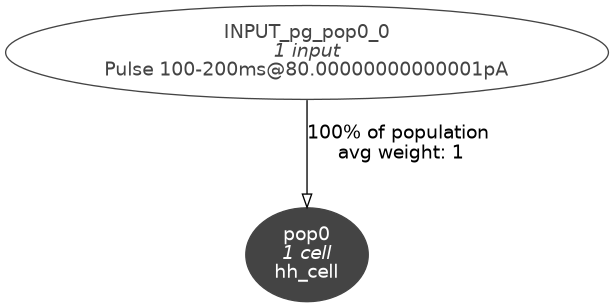Fig. 14 Level 10 network graph generated by pynml#

#### Analysing channels#

Finally, we can analyse the ion channels that we’ve declared using the pynml-channelanalysis utility:

pynml-channelanalysis HH_example_k_channel.nml


This generates graphs to show the behaviour of the channel:Fig. 15 Steady state behaviour of the K ion channel.#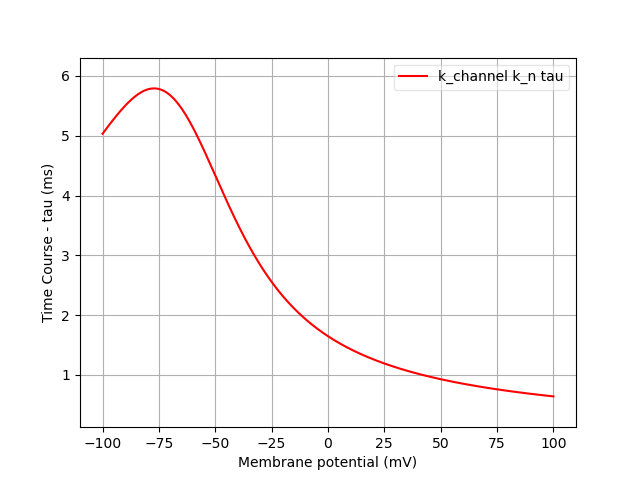Fig. 16 Time course of the K ion channel.#

Similarly, we can get these for the Na channel also:

pynml-channelanalysis HH_example_na_channel.nml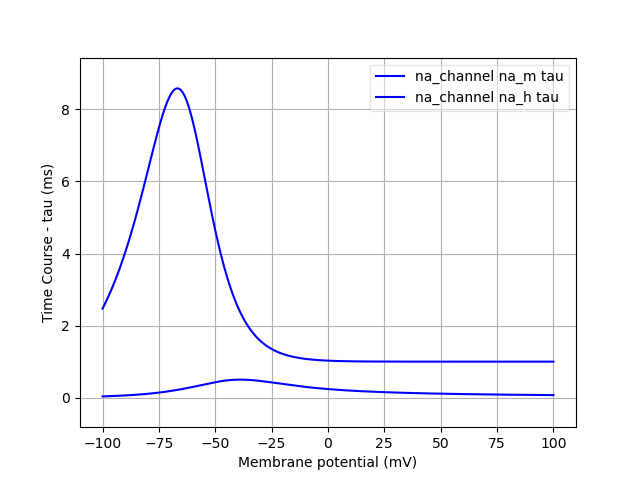Fig. 17 Steady state behaviour of the Na ion channel.#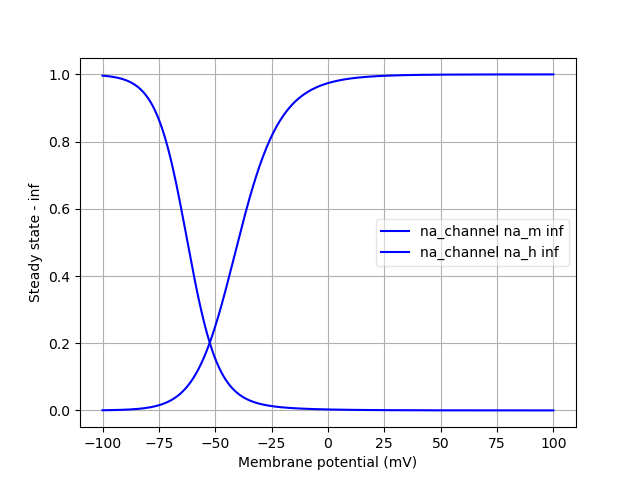Fig. 18 Time course of the Na ion channel.#

## Simulating the model#

Now that we have declared and inspected our network model and all its components, we can proceed to simulate it. We do this in the main function:

def main():
"""Main function

Include the NeuroML model into a LEMS simulation file, run it, plot some
data.
"""
# Simulation bits
sim_id = "HH_single_compartment_example_sim"
simulation = LEMSSimulation(sim_id=sim_id, duration=300, dt=0.01, simulation_seed=123)
# Include the NeuroML model file
simulation.include_neuroml2_file(create_network())
# Assign target for the simulation
simulation.assign_simulation_target("single_hh_cell_network")

# Recording information from the simulation
simulation.create_output_file(id="output0", file_name=sim_id + ".dat")

# Save LEMS simulation to file
sim_file = simulation.save_to_file()

# Run the simulation using the default jNeuroML simulator
pynml.run_lems_with_jneuroml(sim_file, max_memory="2G", nogui=True, plot=False)
# Plot the data
plot_data(sim_id)



Here we first create a LEMSSimulation instance and include our network NeuroML file in it. We must inform LEMS what the target of the simulation is. In our case, it’s the id of our network, single_hh_cell_network:

    sim_id = "HH_single_compartment_example_sim"
simulation = LEMSSimulation(sim_id=sim_id, duration=300, dt=0.01, simulation_seed=123)
# Include the NeuroML model file
simulation.include_neuroml2_file(create_network())
# Assign target for the simulation
simulation.assign_simulation_target("single_hh_cell_network")


We also want to record some information, so we create an output file first with an id of output0:

    simulation.create_output_file(id="output0", file_name=sim_id + ".dat")


Now, we can record any quantity that is exposed by NeuroML (any exposure). For example, we add a column for the membrane potential v of the cell which would be the 0th (and only) cell in our population pop0: pop0/v. We can also record the current in the channels: pop/iChannels We can also record the current density iDensity for the channels, so we also record these.

    simulation.add_column_to_output_file("output0", column_id="pop0/v", quantity="pop0/v")


We then save the LEMS simulation file, run our simulation with the default jNeuroML simulator.

## Plotting the recorded variables#

To plot the variables that we recorded, we read the data and use the generate_plot utility function:

def plot_data(sim_id):
"""Plot the sim data.

Load the data from the file and plot the graph for the membrane potential
using the pynml generate_plot utility function.

:sim_id: ID of simulaton

"""
data_array = np.loadtxt(sim_id + ".dat")
pynml.generate_plot([data_array[:, 0]], [data_array[:, 1]], "Membrane potential", show_plot_already=False, save_figure_to=sim_id + "-v.png", xaxis="time (s)", yaxis="membrane potential (V)")
pynml.generate_plot([data_array[:, 0]], [data_array[:, 2]], "channel current", show_plot_already=False, save_figure_to=sim_id + "-i.png", xaxis="time (s)", yaxis="channel current (A)")
pynml.generate_plot([data_array[:, 0], data_array[:, 0]], [data_array[:, 3], data_array[:, 4]], "current density", labels=["Na", "K"], show_plot_already=False, save_figure_to=sim_id + "-iden.png", xaxis="time (s)", yaxis="current density (A_per_m2)")


This generates the following graphs: